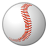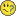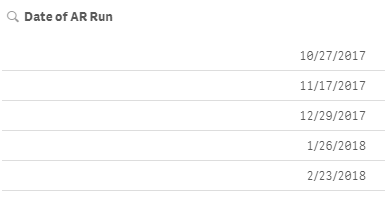# New to Qlik Sense

If you’re new to Qlik Sense, start with this Discussion Board and get up-to-speed quickly.

Announcements
Welcome to Qlik Community! Check out our new navigation! FIND OUT MORE
cancel
Showing results for
Did you mean:Creator

## Current Aging Total Variable

I need help to create a variable for the most current total AR IB Balance. I have the Expression below:

sum({<[Date of AR Run] = {'Max([Date of AR Run])'}>} [Total IB])

But the result is null.

Thanks.

1 Solution

Accepted SolutionsMVP

Try this

Sum({<[Date of AR Run] = {"\$(=Date(Max([Date of AR Run]), 'M/D/YYYY'))"}>} [Total IB])

7 RepliesMVP

Try this

Sum({<[Date of AR Run] = {"\$(=Max([Date of AR Run]))"}>} [Total IB])Specialist

Try to put :

sum({<[Date of AR Run] = {'Date(Max([Date of AR Run]))'}>} [Total IB])

With the same format MM/DD/YYYY for both datesCreator
Author

I tried both, and the the result is Zero now...MVP

What is your date field format?Creator
AuthorMVP

Try this

Sum({<[Date of AR Run] = {"\$(=Date(Max([Date of AR Run]), 'M/D/YYYY'))"}>} [Total IB])Creator
Author

Thanks it worked!Tags
Community Browser